## Friction Questions and Answers Part-6

1. A body of mass 5kg rests on a rough horizontal surface of coefficient of friction 0.2. The body is pulled through a distance of 10m by a horizontal force of 25 N. The kinetic energy acquired by it is $\left(g = 10 m/s^{2}\right)$
a) 330 J
b) 150 J
c) 100 J
d) 50 J

Explanation: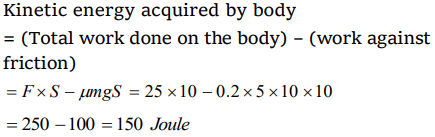2. A motorcycle is travelling on a curved track of radius 500m. If the coefficient of friction between road and tyres is 0.5, the speed avoiding skidding will be
a) 50 m/s
b) 75 m/s
c) 25 m/s
d) 35 m/s

Explanation: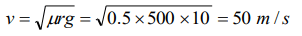3. A block of mass $m=5 kg$  is resting on a rough horizontal surface for which the coefficient of friction is 0.2. When a force $F=40 N$  is applied, the acceleration of the block will be$\left(g=10 m/s^{2}\right)$a) 5.73 $m\diagup s^{2}$
b) 8.0 $m\diagup s^{2}$
c) 3.17 $m\diagup s^{2}$
d) 10.0 $m\diagup s^{2}$

Explanation: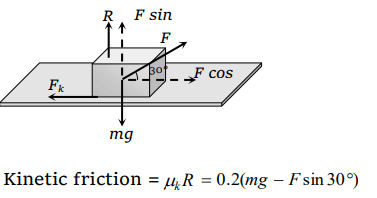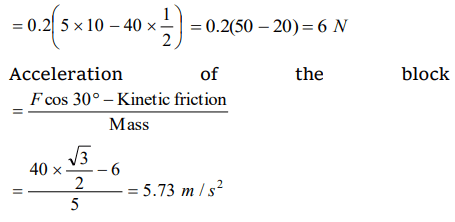4. A body is moving along a rough horizontal surface with an initial velocity 6 m/s. If the body comes to rest after travelling 9 m, then the coefficient of sliding friction will be
a) 0.4
b) 0.2
c) 0.6
d) 0.8

Explanation:5. Consider a car moving on a straight road with a speed of 100 m/s. The distance at which car can be stopped is$\left[\mu_{k}=0.5\right]$
a) 100 m
b) 400 m
c) 800 m
d) 1000 m

Explanation:6. A cylinder of 10 kg is sliding in a plane with an initial velocity of 10 m/s. If the coefficient of friction between the surface and cylinder is 0.5 then before stopping, it will cover. $\left(g = 10 m\diagup s^{2}\right)$
a) 2.5 m
b) 5 m
c) 7.5 m
d) 10 m

Explanation: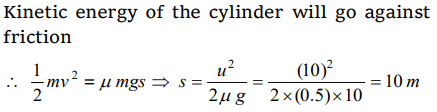7. When a body is lying on a rough inclined plane and does not move, the force of friction
a) is equal to $\mu R$
b) is less than $\mu R$
c) is greater than$\mu R$
d) is equal to R

Explanation: When the body is at rest then static friction works on it, which is less than limiting friction ($\mu$ R).

8. When a body is placed on a rough plane inclined at an angle $\theta$ to the horizontal, its acceleration is
a) $g\left(\sin\theta-\cos\theta\right)$
b) $g\left(\sin\theta-\mu\cos\theta\right)$
c) $g\left(\mu\sin\theta-\cos\theta\right)$
d) $g\mu\left(\sin\theta-\cos\theta\right)$

Explanation: $g\left(\sin\theta-\mu\cos\theta\right)$

9. A block is at rest on an inclined plane making an angle $\alpha$ with the horizontal. As the angle $\alpha$ of the incline is increased, the block starts slipping when the angle of inclination becomes $\theta$. The coefficient of static friction between the block and the surface of the inclined plane is or A body starts sliding down at an angle $\theta$ to horizontal. Then coefficient of friction is equal to
a) $\sin\theta$
b) $\cos\theta$
c) $\tan\theta$
d) Independent of $\theta$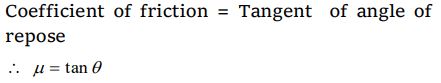a) $\left[1-\frac{1}{n^{2}}\right]$
b) $1-\frac{1}{n^{2}}$
c) $\sqrt{\left[1-\frac{1}{n^{2}}\right]}$
d) $\sqrt{n-\frac{1}{n^{2}}}$